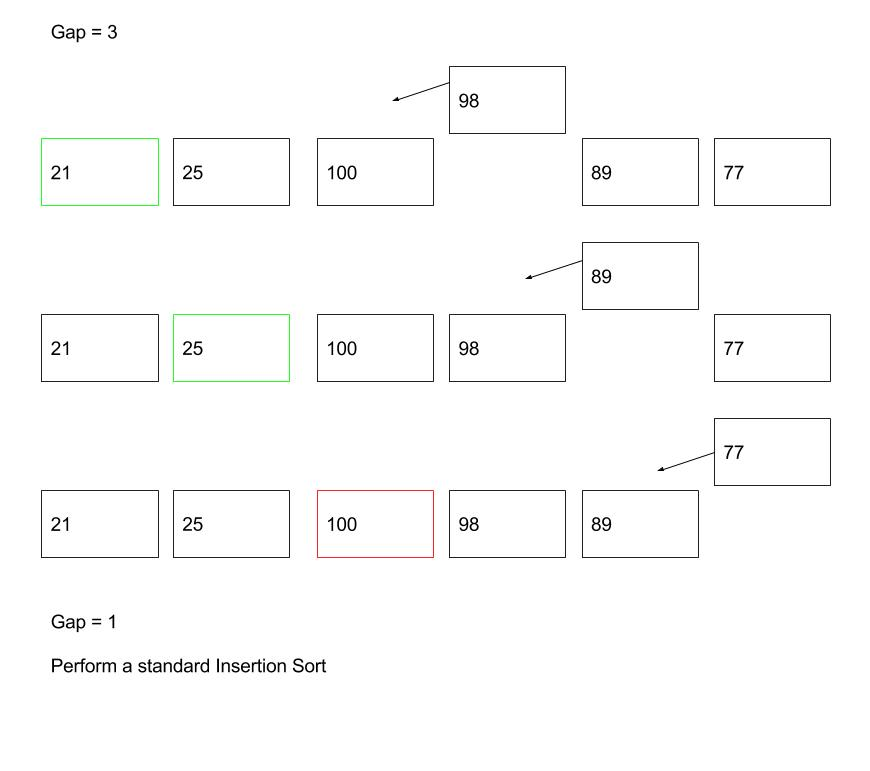# #Data Structure and Algorithm - Shell Sort

In this tutorial, we will learn a simple sorting algorithm - Shell Sort.

## #Problem to Solve

Given a list of numbers as shown below, please sort them in ascending order.

``````\$numbers = [21,25,100,98,89,77];
``````

Requirements:

• You are required to use Shell Sort algorithm when sorting the numbers.
• You are required to implement the algorithm in PHP language.

## #Pseudocode

Shell Sort is a generalisation of Insertion Sort. Unlike Insertion Sort, instead of comparing contiguous items, it breaks the master list into several sub-lists using an interval i (which is known as the gap), then sorts the sub-lists with Insertion Sort.

We repeat the steps above until the gap becomes 1, then we essentially apply a standard Insertion Sort.

Apparently, the gap sequence plays an important role in this sort algorithm. Unfortunately, there is no perfect gap sequence, so to speak. Different researchers have come out a couple of different gap sequences, their performance depends heavily on the input data size.

Some popular gap sequences:

• Shell Sequence: FLOOR(N/2k)
• Pratt Sequence: 2p3q
• Knuth Sequence: (3k â€“ 1) / 2

We are using Shell Sequence in this tutorial.Pseudocode of Shell Sort algorithm can be written as follows:

``````Caculate gap size (\$gap)

WHILE \$gap is greater than 0

FOR each element of the list, that is \$gap apart

Extract the current item

Locate the position to insert

Insert the item to the position

END FOR

Calculate gap size (\$gap)

END WHILE
``````

## #PHP Implementation

We need a FOR loop and a WHILE loop. We are using the FOR loop to iterate the master list and the WHILE loop to locate the position to insert the item.

``````<?php
\$numbers = [21, 25, 100, 98, 89, 77];

\$gap  = floor(count(\$numbers)/2);

while (\$gap > 0) {

for (\$i = 0; \$i < count(\$numbers) - \$gap; \$i++) {

\$extractItem = \$numbers[\$i + \$gap];

\$positionFound = \$i + \$gap;

while ((\$positionFound - \$gap) > 0 && \$extractItem < \$numbers[\$positionFound - \$gap]) {

\$numbers[\$positionFound] = \$numbers[\$positionFound - \$gap];

\$positionFound = \$positionFound - \$gap;
}

\$numbers[\$positionFound] = \$extractItem;
}

\$gap = floor(\$gap/2);
}

print_r(\$numbers);

// Output:
/*
Array
(
 => 21
 => 25
 => 77
 => 89
 => 98
 => 100
)
*/
``````

The implementation is similar to Insertion Sort, except that we are comparing items that are \$gap apart until the \$gap values become 1. Then we perform a standard Insertion Sort.